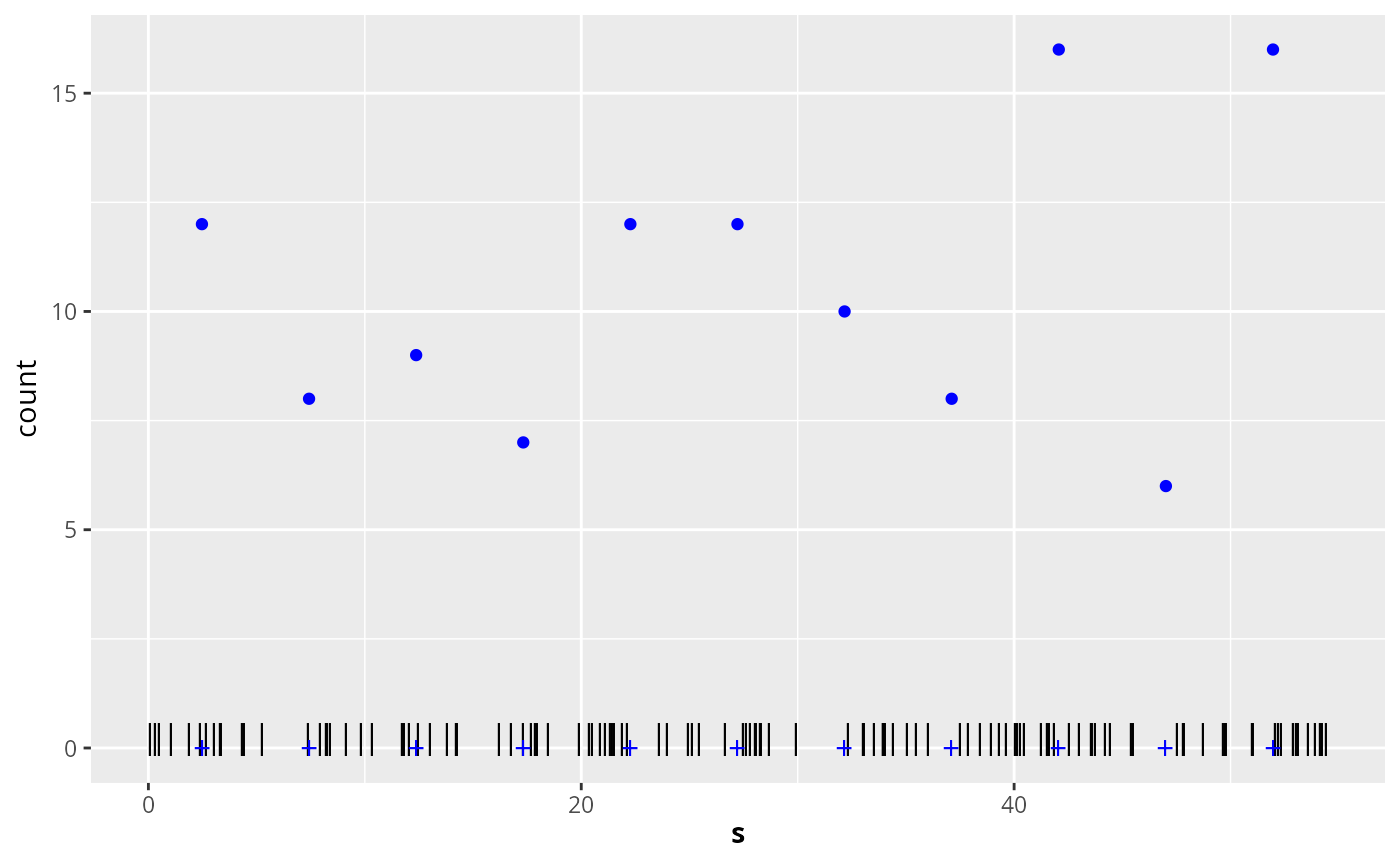Point data and count data, together with intensity function and expected counts for a homogeneous 1-dimensional Poisson process example.

## Usage

data(Poisson1_1D)

## Format

The data contain the following R objects:

lambda1_1D:

A function defining the intensity function of a nonhomogeneous Poisson process. Note that this function is only defined on the interval (0,55).

E_nc1

The expected counts of the gridded data.

pts1

The locations of the observed points (a data frame with one column, named x).

countdata1

A data frame with three columns, containing the count data:

## Examples

# \donttest{
if (require("ggplot2", quietly = TRUE)) {
data(Poisson1_1D)
ggplot(countdata1) +
geom_point(data = countdata1, aes(x = x, y = count), col = "blue") +
ylim(0, max(countdata1\$count)) +
geom_point(data = pts1, aes(x = x), y = 0.2, shape = "|", cex = 4) +
geom_point(
data = countdata1, aes(x = x), y = 0, shape = "+",
col = "blue", cex = 4
) +
xlab(expression(bold(s))) +
ylab("count")
}# }